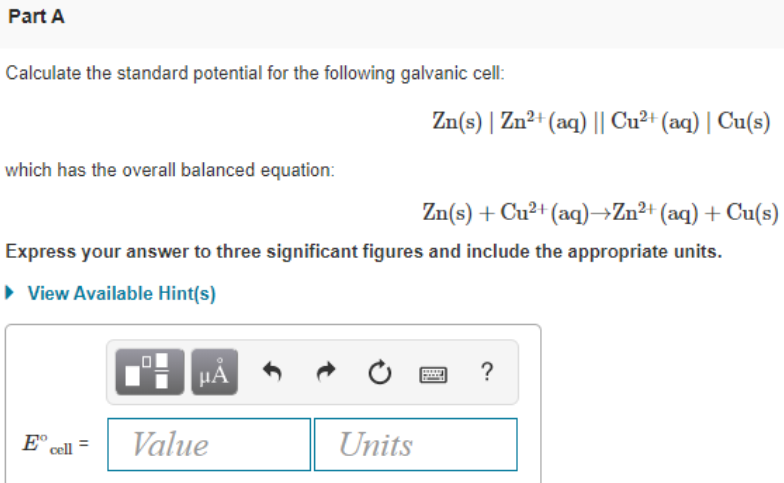# Calculate the standard potential for the following galvanic cell: Zn(s) / Zn^2+(aq) // Cu^2+(aq) / Cu(s) which has the overall balanced equation: Zn(s) + Cu^2+(aq) → Zn^2+(aq) + Cu(s) Express your answer to three significant figures and include the appropriate units.